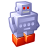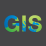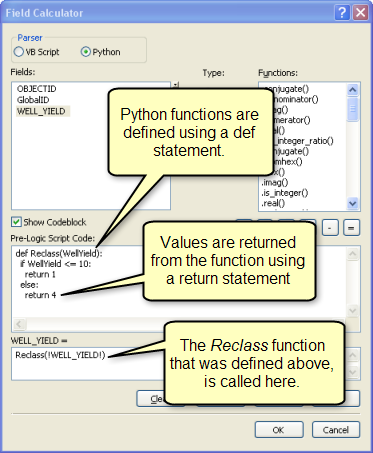# Python script/expression for field calculator for converting bearings to directions?

4971
27
09-22-2016 10:03 AMNew Contributor II

I have a double field with bearings/azimuths (numbers) and I need them in letter directions: N, NE, NW,etc. I would like to calculate them in  a new field.  I have searched high and low for the code to use in the field calculator but I have failed to figure it out.

Thanks,

Bob

1 Solution

Accepted SolutionsbyMVP Esteemed Contributor

``````import numpy as np
global a
global c
a = np.array([ 45., 135., 225., 315., 360])
c = np.array(['N', 'E', 'S', 'W', 'N'])
def compass(angle):
""" test """
return c[np.digitize([angle], a)]
‍‍‍‍‍‍‍‍``````

useage

``````compass(!YourFieldNameHere!)  # using the python processor of course
‍``````

although I hate to use globals.

Now when you do this in python (TableToNumPyArray or FeatureClassToNumPyArray) you can get the results quickly or as a demo script

``````    angles = [0, 44, 46, 134, 136, 224, 226, 314, 316, 359]
for i in angles:
print("{} => {}".format(i, compass(i)))‍‍‍``````

Yielding...

``````0 => ['N']
44 => ['N']
46 => ['E']
134 => ['E']
136 => ['S']
224 => ['S']
226 => ['W']
314 => ['W']
316 => ['N']
359 => ['N']‍‍‍‍‍‍‍‍‍‍``````

Why use this?  Because the next thing is that someone will want to narrow down things into 22.5 degree chunks (ie NNW or NNE etc etc)

To change the above, you just need to fiddle in some more compass bearings and compass designators.

27 RepliesbyEsri Esteemed Contributor

Hi Bob,

If the lines represent the bearings/azimuth correctly you can add COGO fields to the feature class and then update the COGO attributes.New Contributor II

Thanks,

I am not working with points. I think I am in need of an if/else python

script.

BobbyMVP Regular Contributor

The COGO stuff Jake Skinner‌ posted is worth looking into. If you just want something quick and custom, could expand on logic like this?

``````if in_field_val >= 0 and in_field_val < 90:
out_dir = "N"
elif in_field_val >= 90 and in_field_val < 180:
out_dir = "E"
elif in_field_val >= 180 and in_field_val < 270:
out_dir = "S"
elif in_field_val >= 270 and in_field_val < 360:
out_dir = "W"
else:
out_dir = None‍‍‍‍‍‍‍‍‍‍``````New Contributor II

Blake,

so, I don't need to check off the code block in field calculator, i.e., I

don't need the pre-logic script code and the other part?

thanks,

BobbyMVP Esteemed Contributor

when you are using a code block, then you need to show the code block just like in the picture

Calculate Field examples—Help | ArcGIS for Desktop in the code block section.

I use python as everyone will at some stagebyMVP Esteemed Contributor

``````import numpy as np
global a
global c
a = np.array([ 45., 135., 225., 315., 360])
c = np.array(['N', 'E', 'S', 'W', 'N'])
def compass(angle):
""" test """
return c[np.digitize([angle], a)]
‍‍‍‍‍‍‍‍``````

useage

``````compass(!YourFieldNameHere!)  # using the python processor of course
‍``````

although I hate to use globals.

Now when you do this in python (TableToNumPyArray or FeatureClassToNumPyArray) you can get the results quickly or as a demo script

``````    angles = [0, 44, 46, 134, 136, 224, 226, 314, 316, 359]
for i in angles:
print("{} => {}".format(i, compass(i)))‍‍‍``````

Yielding...

``````0 => ['N']
44 => ['N']
46 => ['E']
134 => ['E']
136 => ['S']
224 => ['S']
226 => ['W']
314 => ['W']
316 => ['N']
359 => ['N']‍‍‍‍‍‍‍‍‍‍``````

Why use this?  Because the next thing is that someone will want to narrow down things into 22.5 degree chunks (ie NNW or NNE etc etc)

To change the above, you just need to fiddle in some more compass bearings and compass designators.New Contributor II

Isn't a if/else statement possible?

I need to look this over more.byMVP Esteemed Contributor

you copy the first block i posted just like you would any function in the codeblock area (including all lines 1-8)

Then I gave you the expression box syntax (make sure you use the python parser in the field calculator), and replace the !YourFieldNameHere! with your field name surrounded by exclamation marks..

The other two blocks are just demo scripts I used on my iThingy  with Pythonista version 3.5, but it should still work with python 2.7byMVP Honored Contributor

Very similar to other solutions that could be adapted to the field calculator:

Python parser

Expression:

``myfunc( !bearing! )‍``

Codeblock:

``````dict = {'N':[0,90],'E':[90,180],'S':[180,270],'W':[270,360]}
def myfunc(bearing):
for k,v in dict.iteritems():
if bearing >= v and bearing < v:
return k‍‍‍‍‍``````